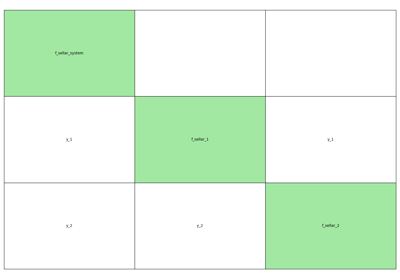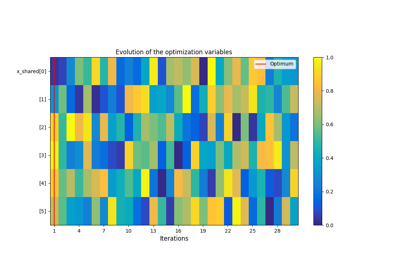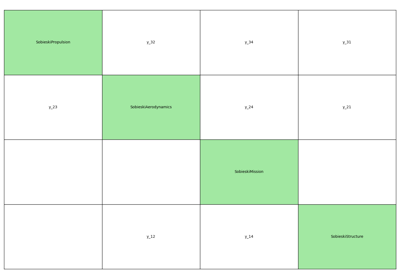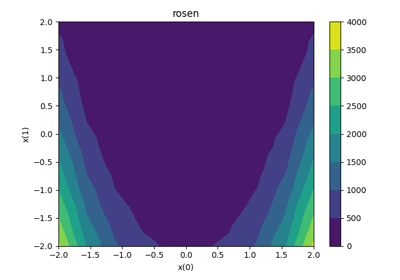gemseo / datasets

# optimization_dataset module¶

A Dataset to store optimization histories.

class gemseo.datasets.optimization_dataset.OptimizationDataset(data=None, index=None, columns=None, dtype=None, copy=None, *, dataset_name='')[source]

Bases: Dataset

A Dataset to store optimization histories.

Warning

A Dataset behaves like any multi-index DataFrame but its instantiation using the constructor dataset = Dataset(data, ...) can lead to some inconsistencies (multi-index levels, index values, dtypes, …). Hence, the construction from the dedicated methods is recommended, e.g. dataset = Dataset(); dataset.add_variable("x", data).

Notes

The columns of a data structure (NumPy array, DataFrame, Dataset, …) are called features. The features of a Dataset include all the components of all the variables of all the groups.

Initialize self. See help(type(self)) for accurate signature.

Parameters:
• dataset_name (str) –

The name of the dataset.

By default it is set to “”.

• data (ndarray | Iterable | dict | DataFrame | None) –

• index (Axes | None) –

• columns (Axes | None) –

• dtype (Dtype | None) –

• copy (bool | None) –

Add the data related to the constraint group.

Parameters:
• data (DataType) – The data.

• variable_names (StrColumnType) –

The names of the variables. If empty, use DEFAULT_VARIABLE_NAME.

By default it is set to ().

• variable_names_to_n_components (dict[str, int] | None) – The number of components of the variables. If variable_names is empty, this argument is not considered. If None, assume that all the variables have a single component.

Return type:

None

Add data related to a constraint.

Parameters:
• variable_name (str) – The name of the variable.

• data (ndarray | Iterable[Any] | Any) – The data, either an array shaped as (n_entries, n_features), an array shaped as (n_entries,) that will be reshaped as (n_entries, 1) or a scalar that will be converted into an array shaped as (n_entries, 1).

• components (int | Iterable[int]) –

The components considered. If empty, use [0, ..., n_features].

By default it is set to ().

Return type:

None

Add the data related to the design variable group.

Parameters:
• data (DataType) – The data.

• variable_names (StrColumnType) –

The names of the variables. If empty, use DEFAULT_VARIABLE_NAME.

By default it is set to ().

• variable_names_to_n_components (dict[str, int] | None) – The number of components of the variables. If variable_names is empty, this argument is not considered. If None, assume that all the variables have a single component.

Return type:

None

Add data related to a design variable.

Parameters:
• variable_name (str) – The name of the variable.

• data (ndarray | Iterable[Any] | Any) – The data, either an array shaped as (n_entries, n_features), an array shaped as (n_entries,) that will be reshaped as (n_entries, 1) or a scalar that will be converted into an array shaped as (n_entries, 1).

• components (int | Iterable[int]) –

The components considered. If empty, use [0, ..., n_features].

By default it is set to ().

Return type:

None

Add the data related to the objective group.

Parameters:
• data (DataType) – The data.

• variable_names (StrColumnType) –

The names of the variables. If empty, use DEFAULT_VARIABLE_NAME.

By default it is set to ().

• variable_names_to_n_components (dict[str, int] | None) – The number of components of the variables. If variable_names is empty, this argument is not considered. If None, assume that all the variables have a single component.

Return type:

None

Add data related to an objective.

Parameters:
• variable_name (str) – The name of the variable.

• data (ndarray | Iterable[Any] | Any) – The data, either an array shaped as (n_entries, n_features), an array shaped as (n_entries,) that will be reshaped as (n_entries, 1) or a scalar that will be converted into an array shaped as (n_entries, 1).

• components (int | Iterable[int]) –

The components considered. If empty, use [0, ..., n_features].

By default it is set to ().

Return type:

None

Add the data related to the observable group.

Parameters:
• data (DataType) – The data.

• variable_names (StrColumnType) –

The names of the variables. If empty, use DEFAULT_VARIABLE_NAME.

By default it is set to ().

• variable_names_to_n_components (dict[str, int] | None) – The number of components of the variables. If variable_names is empty, this argument is not considered. If None, assume that all the variables have a single component.

Return type:

None

Add data related to an observable.

Parameters:
• variable_name (str) – The name of the variable.

• data (ndarray | Iterable[Any] | Any) – The data, either an array shaped as (n_entries, n_features), an array shaped as (n_entries,) that will be reshaped as (n_entries, 1) or a scalar that will be converted into an array shaped as (n_entries, 1).

• components (int | Iterable[int]) –

The components considered. If empty, use [0, ..., n_features].

By default it is set to ().

Return type:

None

CONSTRAINT_GROUP: Final[str] = 'constraints'

The group name for the constraints of an OptimizationProblem.

DESIGN_GROUP: Final[str] = 'designs'

The group name for the design variables of an OptimizationProblem.

FUNCTION_GROUP: Final[str] = 'functions'

The group name for the functions of an OptimizationProblem.

OBJECTIVE_GROUP: Final[str] = 'objectives'

The group name for the objectives of an OptimizationProblem.

OBSERVABLE_GROUP: Final[str] = 'observables.'

The group name for the observables of an OptimizationProblem.

property constraint_dataset: OptimizationDataset

The view of the constraint dataset.

property constraint_names: list[str]

The names of the constraints.

property design_dataset: OptimizationDataset

The view of the design dataset.

property design_variable_names: list[str]

The names of the design variables.

property iterations: list[int]

The iterations.

misc: dict[str, Any]

Miscellaneous information specific to the dataset, and not to an entry.

property n_iterations: int

The number of iterations.

name: str

The name of the dataset.

property objective_dataset: OptimizationDataset

The view of the objective dataset.

property objective_names: list[str]

The names of the objectives.

property observable_dataset: OptimizationDataset

The view of the observable dataset.

property observable_names: list[str]

The names of the observables.

## Examples using OptimizationDataset¶Parameter space

Parameter spaceCreate an MDO Scenario

Create an MDO ScenarioGEMSEO in 10 minutes

GEMSEO in 10 minutesBiLevel-based DOE on the Sobieski SSBJ test case

BiLevel-based DOE on the Sobieski SSBJ test caseMDF-based DOE on the Sobieski SSBJ test case

MDF-based DOE on the Sobieski SSBJ test caseThe optimisation dataset

The optimisation datasetZvsXY

ZvsXY